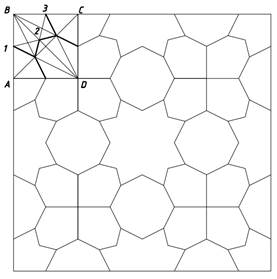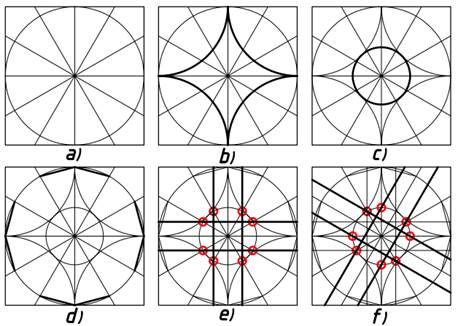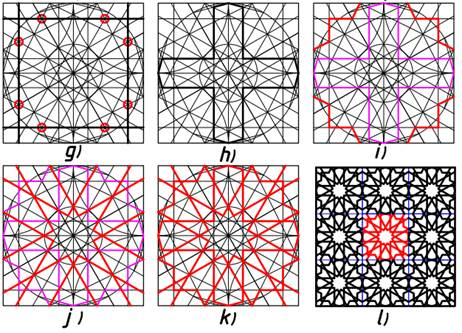# GRAPHIC RECOMMENDATIONS FOR THE ANALYSIS OF SIMPLE AND COMPLEX GIRIX PATTERN COMPOSITIONS IN THE ART OF EMBROIDERY BY GEOMETRIC PATTERNS

ГРАФИЧЕСКИЕ РЕКОМЕНДАЦИИ ПО АНАЛИЗУ ПРОСТЫХ И СЛОЖНЫХ КОМПОЗИЦИЙ ГИРИКСОВЫХ УЗОРОВ В ИСКУССТВЕ ВЫШИВКИ ГЕОМЕТРИЧЕСКИМИ УЗОРАМИ
Цитировать:
Sattarov S.Y., Xaitov Z.A., Yusubova M.A. GRAPHIC RECOMMENDATIONS FOR THE ANALYSIS OF SIMPLE AND COMPLEX GIRIX PATTERN COMPOSITIONS IN THE ART OF EMBROIDERY BY GEOMETRIC PATTERNS // Universum: технические науки : электрон. научн. журн. 2021. 11(92). URL: https://7universum.com/ru/tech/archive/item/12606 (дата обращения: 28.09.2023).
Прочитать статью:

ABSTRACT

In this article, graphic recommendations for the analysis of simple and complex girix pattern compositions in the art of embroidery by geometric patterns are given.

АННОТАЦИЯ

В этой статье приведены графические рекомендации по анализу простых и сложных композиций гирих-узоров в нейл-арте посредством геометрических рисунков.

Keywords: Patterns, girix embroidery, composition, architecture, painting, drawing, shapes.

Ключевые слова: Узор, гироскоп, композиция, архитектура, рисунок, рисунок, формы.

Girix forms the basis of the pattern composition by methods of dividing the cross-section or circle into mutually equal or propotsional pieces. Suppose you need to divide the circle into equal parts in a box. In the bun, a thread equal to the length of the circle is obtained, with the help of twisting it, the given circle is cut into pieces, and the arcs of the circle are obtained equal to those pieces. Here it is possible to make a cross section of a straight line and a circle by the method described above, before it is known the number of equal parts among themselves. The ancient period of making such a pattern (land. Known since the previous 4-2 thousand years). And the division of circles into equal pairs of pieces with high accuracy corresponds to the heads of the second half of the 1 thousand years before the era.

In subsequent periods, we see the distribution of the circle into any pieces without a remainder equal to three, in connection with the “Golden distribution (cross section)” of the circles of the circle.

It is known that the so-called Golden Ratio is widely used in the analysis of the geometrical principles of such Sciences as plastic Anatomy, architecture, aesthetics. One side of the drawing paper formats according to the state standard is determined on the basis of the ratio in the same “golden section” relative to the second side. In subsequent periods, we see that the division of the cross-section, circles into equal or proportional parts is applied to more complex works. For example, in Fakhir Ali girihi, which dates back to the 14th century in Samarkand, the execution of the drawing was used to divide the complex circle into seven equal parts.

Girix pattern is made mainly on the basis of geometrical patterns. The pattern can be inserted into the path, Circle, Square and various polygons. Types of patterns are diverse, knowledge about which can be obtained through special literature. Bunda is made from straight lines “girix”, that is, from the simplest view of a complex pattern called a knot, to the most familiar of the complex ones (Pictures 1-a, b, d, e, f, g).

1. A square is drawn and its diagonals are held. Through the middle of the square draw the center lines. Draw a circle that will move to the sides of the square (Figure 1, a).

2. At 1, 2, 3, 4 points intersecting a circle with Square diagonals, draw arcs of a circle that intersect (Figure 1, b).

3. On the sides of the square, points A, B, D, S are intersected by 5, 6, 7, 8 points, making a regular Octagon (Figure 1, d).

4. Continuing with the sides of the formed regular Octagon, draw a pattern, like in Figure 1, e, that is, girix.Figure 1. Patterns

5. On both sides of the pattern lines, auxiliary lines of a certain size are transferred (Figure 1, f).

6. The elements of the pattern are “clipped” so that they intersect each other (Figure 1, g).

Simply assemble. We comb the Square from point D with three Rays. From point B we also comb three light bulbs. We pass the AC diagonal. The first nurchizik, combed from D, dates AB at 1 point. With B, we find 2 and 3 points by drawing an arc from B with a radius of 1 point spacing. We connect the 2 point with the point where the first and third Rays intersect diagonally. Then we smear the lines that make the girix. (Figure 2).Figure 2. The lines that make the girix

Make complex graphs. Girix patterns are made on the basis of geometrical patterns, as we have already described above. Elements of a geometric pattern consist of four parts – triangles, rectangles, polygons and curved lines. It is possible to make thousands of patterns by comparing and placing the same girix elements among themselves. From the repetition of the distributions, a complete pattern composition is formed. There are specific difficulties in drawing up a geometric pattern composition. To do this, a good knowledge of mathematics and geometry is required. Below is a link to a way to compose a new girix composition.

1. A square is drawn, and through its middle draw the center lines. Draw an inner circle, which will try on the sides of the square. Then the circle is divided into equal 12. Draw an arc with a radius equal to half the sides of the square. (Figure 3, a, b).

2. Urin try on the arcs drawn from the sides of the square draw an inner circle. Then two (large and small) circles are formed inside the square. A straight line is drawn that intersects the 3 point of the intersection of the lines that make up the circle with the circle. We connect the 3 point of the circle and leave the 2 point in the middle without fading. As a result, an appearance is formed in the following drawing. (Figure 3, c, d).

3. Parallel to the two sides of the center lines dividing the square into four, draw horizontal and vertical lines. These horizontal and vertical lines pass through the intersection point of the lines dividing the inner small circle and the circle into parts. (These intersection points are marked by a Red Circle Line). Then again, a parallel line is drawn on the incline, intersecting the inner small circle and the lines dividing the circle into equal parts from the intersection point. Parallel lines drawn on the incline will also be parallel to the curvature line, dividing the circle into equal parts. (These intersection points are marked by a Red Circle Line). (Figure 3, e, f).

4. Parallel lines drawn horizontally, vertically and obliquely go, and from the points that are connected to the square, intersect each other with lines. As a result, a picture reminiscent of an 8-pointed star image is formed. Then draw parallel lines inside the sides of the square. These parallel lines intersect with lines that divide the circle into equal parts and intersect the formed edge ends with adjacent lines. (These intersection points are marked by a Red Circle Line). In the next step, we connect the vertical and horizontal parallel lines, dividing the circle into equal parts, the end between the sides of the square. (The picture below shows a red line). (Figure 3, g, h, i)

5. Now the lines formed by the edge are drawn as shown in the figure below, separated by a red line. After the edges are formed, parallel oblique lines are drawn to the lines dividing the circle into equal parts. (Figure 3, j).Figure 3. Patterns

6. After all the auxiliary lines are drawn, the main creamy lines that make the girix are drawn, making it creamy. Auxiliary lines are erased and girix looks holistic. Gazebsizki girix was ready. This is a fraction of girix, and from this fraction a holistic composition is formed, dissolving girix. (Figure 3, k, l)

In conclusion, it can be said that drawing (drawing) the role of drawing in the development of the consciousness of mankind, in the imagination of its surrounding being and in the development of a great blessing, which is called Thinking by bringing its own understanding to another, is extremely important. In this regard, when composing girix pattern compositions, the role and practical significance of geometrical patterns is considered important.

Indeed, if each girix is drawn on the basis of elements of geometrical construction, before drawing it is simple, complex, then the girix under drawing will have a clear solution. Before compiling each girix, which consists of a solution, geometric fabrication elements are consulted.

Well, it turns out that the development of mankind and society is closely connected with the drawings. But through the process of studying the environment, that is, existence, people are engaged in science in the interests of their own benefit, in the interests of their native land, in the interests of the world and open new discoveries. To bring all this to the people of the world, the most first of all is addressed to the drawings.

List of used literature:

1. S.S. Bulatov, P.P. Shabaratov, M.A. Rasulov “Naqqoshlik” Toshkent “Iqtisod-moliya” 2010 4. S.S.Bulatov. “O‘zbek xalq amaliy san’ati bezagi” - T.: “Mexnat” 1991.
2. Mohammad C., Abdugafforovich T. K. Fundamentals and standards of design of a modern fuel station building //Academicia Globe: Inderscience Research. – 2021. – Т. 2. – №. 05. – С. 431-435.
3. Mustafa M. A., Abdugafforovich T. K. The role of urban planning norms and rules in creating a modern bank building design with graphic software //Innovative Technologica: Methodical Research Journal. – 2021. – Т. 2. – №. 05. – С. 143-146.
4. Seerat M., Abdugafforovich T. K. Theoretical and methodical instructions for the implementation of the modern project of the building of the place of storage of food products. – 2021.
5. Tokhirov A. Using the graphical editor "компас 3d"  in teaching computer engineering graphics. Universum: technical sciences: a scientific journal. - No. 7 (88). Part 3.M., Ed. "MCNO", 2021. - 84 p. - Electron. print version publ. http://7universum.com/ru/tech/archive/category/788 DOI: 10.32743/UniTech.2021.78.8-3.12076
6. Tokhirov A. Application procedure cad / cam / cae  systems in scientific research, Universum: technical sciences: a scientific journal. - No. 6 (87). Part 5.M., Ed. "MCNO", 2021. - 72 p. - Electron. print version publ. http://7universum.com/ru/tech/archive/category/687, DOI - 10.32743/UniTech.2021.87.6.11836
Информация об авторахTeacher of the Department of Fine Arts and engineering graphics, TerSU, Uzbekistan, Termez

преподаватель кафедры изобразительного искусства и инженерной графики, Термезский государственный университет, Узбекистан, г. ТермезTeacher of the Department of Fine Arts and engineering graphics, TerSU, Uzbekistan, Termez

преподаватель кафедры изобразительного искусства и инженерной графики, Термезский государственный университет, Узбекистан, г. ТермезStudent of the direction of Fine Arts and engineering graphics, TerSU, Uzbekistan, Termez

студент направления Изобразительное искусство и инженерная графика, Термезский государственный университет, Узбекистан, г. Термез

Журнал зарегистрирован Федеральной службой по надзору в сфере связи, информационных технологий и массовых коммуникаций (Роскомнадзор), регистрационный номер ЭЛ №ФС77-54434 от 17.06.2013
Учредитель журнала - ООО «МЦНО»
Главный редактор - Ахметов Сайранбек Махсутович.
Top